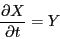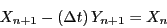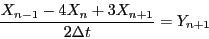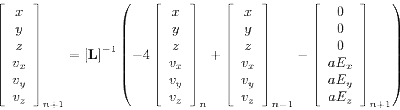## Wednesday, October 22, 2008

### Planar Magnetron

A magnetron is a device useful for generating lots of microwave radiation, most homes have magnetron's which use roughly 1kW of microwave power for heating food. A magnetron acts like a diode, when the magnetic field between the inner cathode and the outer anode reaches a certain level, electrons starting at the cathode will not be able to reach the anode. This level of magnetic field is known as the Hull cut-off magnetic field of the magnetron.

Suppose we have a simple planar magnetron with constant E and B fields. The path taken by an electron starting from rest at the cathode is governed by the relation of the time rate of change of the particle to the forces on the particle caused by the E and B fields.Assuming constant particle mass and neglecting relativistic effects gives an equation for particle acceleration:This second order differential equation can be recast as a system of first order equations:Where X is the vector of position and velocity components given byAnd Y is the vector of velocity and acceleration components given byThe initial conditions are X = 0 andThis system can be integrated numerically with the explicit forward Euler method:The bisection method is used to find the value of B that causes a maximum of 1 cm
displacement in the y-direction. This 1 cm-high path taken by the electron with Bz = 33.7884
Gauss and Ey = −1/3 stat-volt/cm is shown in the figure below.
Explicit Integration:Alternatively, an implicit backward Euler method can be used:The left hand side can be consolidated into a single operator, L, acting on the unkown vector at the future time-step and the additive term due to the electric field:This operator must be inverted, this can be done once and then each successive time-step
can be calculated with a matrix-vector multiply.First Order Implicit Integration:Using a second order accurate approximation for the first derivative (see finite difference for their relationship with derivatives) provides a more accurate solution, and makes the solution for the larger time-step sizes more reasonable:Collecting all of the n + 1 terms as we did before, givesOur implicit integration operator then just has a few slight modifications, and the right hand side now includes n and n − 1 terms.Second Order Implicit Integration:The second order method achieves something closer the "correct" shape much more quickly than the first-order scheme.

This analysis ignores any losses due to radiation from the accelerated particle (which of course is why magnetrons are useful in the first place).

A pdf and various Octave/Python/Maxima scripts for this problem are on:
1.2.